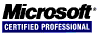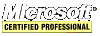## Bubble Sort Algorithmn in C/C++

If an array is already sorted and the first element is changed then there is no faster algorithm than this one to re-sort the array. If an element in the middle or near the end is changed to a smaller value making it belong near the top then bubble sort will perform very poorly because the value that is out of place must bubble up with each pass of the algorithm.

The `bubble_sort_optimized` algorithm fixes this shortcoming by attacking the array from both ends. The idea is to lessen the bubbling action. The more random the array then the more bubbling that will occur. This algorithm is only efficient when there are a few elements out of place of an otherwise sorted array. So good use of this algorithm would be a situtation where there is a sorted array that must have a value changed/edited; call this algorithm and it will quickly re-sort the array. Alternatively, determine if the changed value is lessened or greatened and then choose either `bubble_sort` or `bubble_sort_reversed` to resort the array.

The `bubble_sort_reversed` algorithm is useful after adding an item to the end of an array, for example.

See the Algorithm Study for more details.

``````// the original algorithm
void bubble_sort(int array[], const int size){
int keepswapping; int temp;
do{
keepswapping = false;
for(int count = 0; count < (size - 1); count++)
if(array[count] > array[count + 1]){
temp = array[count];
array[count] = array[count + 1];
array[count + 1] = temp;
keepswapping = true;
}
}while(keepswapping);
}

void bubble_sort_reversed(int array[], const int size){
int keepswapping; int count, temp;
do{
keepswapping = false;
for(count = size-1; count > 0; count--)
if(array[count] < array[count - 1]){
temp = array[count];
array[count] = array[count - 1];
array[count - 1] = temp;
keepswapping = true;
}
}while(keepswapping);
}

// if there are only one or two items that are out of order in the
// array then there is nothing faster than this algorithm
void bubble_sort_optimized(int array[], const int size){
int keepswapping; int count, temp; const int end = size-1;
do{
for(count = end; count > 0; count--)
if(array[count] < array[count - 1]){
temp = array[count];
array[count] = array[count - 1];
array[count - 1] = temp;
}
keepswapping = false;
for(count = 0; count < end; count++)
if(array[count] > array[count + 1]){
temp = array[count];
array[count] = array[count + 1];
array[count + 1] = temp;
keepswapping = true;
}
}while(keepswapping);
}

void bubble_sort_optimized_string(char *array[], const int size){
int keepswapping; int count; char *temp; const int end = size-1;
do{
for(count = end; count > 0; count--)
if(_stricmp(array[count], array[count - 1]) < 0){
temp = array[count];
array[count] = array[count - 1];
array[count - 1] = temp;
}
keepswapping = false;
for(count = 0; count < end; count++)
if(_stricmp(array[count], array[count + 1]) > 0){
temp = array[count];
array[count] = array[count + 1];
array[count + 1] = temp;
keepswapping = true;
}
}while(keepswapping);
}

``````
```   Profile Add comment Sign in ```
``` HTML/CSS JavaScript C/C++ .NET Xamarin Assembly PHP IT © 2020 - PROWARE tech. privacy policy ```
``` ```
``` ```